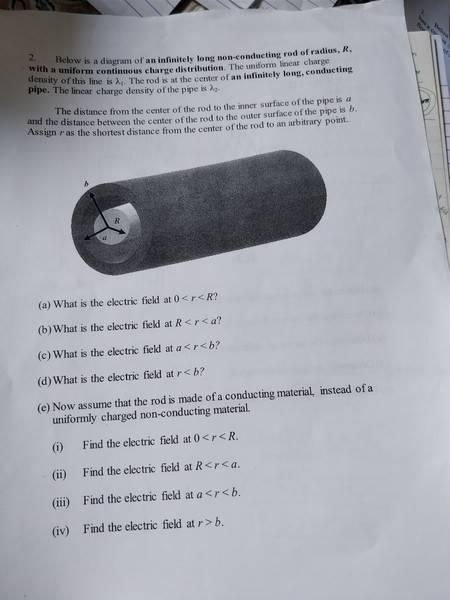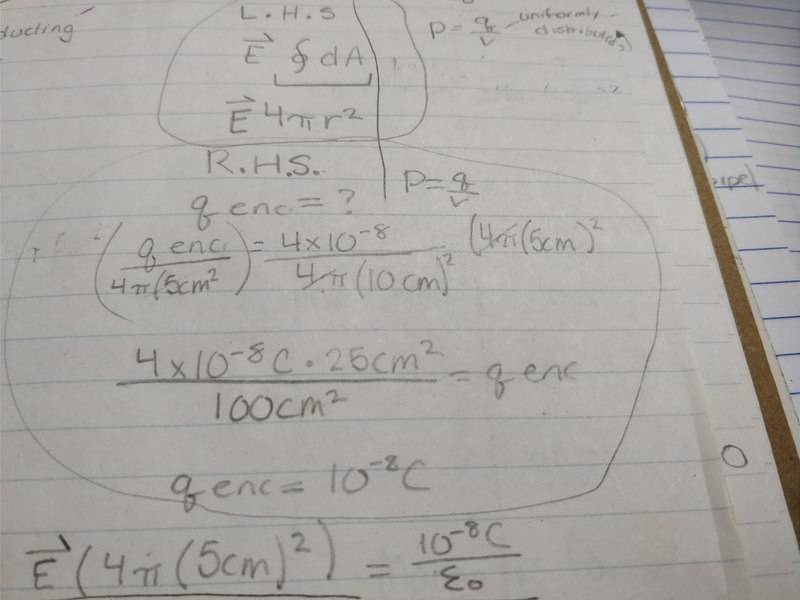# Gauss Law- Conducting and Non-conducting cylindrical shells

Samnolan1031

## Homework StatementBelow is a diagram of an infinitely long non-conducting rod of radius, R, with a uniform continuous charge distribution. The uniform linear charge density of this line is lamba1. The rod is at the center of an infinitely long, conducting pipe. The linear charge density of the pipe is lamba2.
The distance from the center of the rod to the inner surface of the pipe is a and the distance between the center of the rod to the outer surface of the pipe is b. Assign r as the shortest distance from the center of the rod to an arbitrary point.
a) what is the electric field at 0<r<R
b) what is the electric field at R<r<a?
c) what is the electric field at a<r<b?
d) what is the electric field at r<b?

## Homework Equations

Gauss's Law:[/B]
integral of E*da=qenc/Eo
Density of lamba 1=Q/L
Density of lamba 2=Q/V

## The Attempt at a Solution

a) E*dA=qenc/Eo
E*(2pirL)=lamba1*L/Eo
E=lamba1*L/2pi*r*L*Eo
E=lamba1/2pi*r*Eo

b) the answer would the same as answer a because it is asking for the hollow space.
c) the electric field would be 0 because the pipe is conducting.
d)
E*dA= (E1+E2)/Eo
E= (E1+E2)/Eo * 1/2pir^2

Are my answers correct so far? Thank you!

Homework Helper
Gold Member

## The Attempt at a Solution

a) E*dA=qenc/Eo
E*(2pirL)=lamba1*L/Eo
No; remember that the inner rod has uniform charge density. So what will be Q(r)?
E=lamba1*L/2pi*r*L*Eo
E=lamba1/2pi*r*Eo
The E field is not infinite at r=0 is it?
b) the answer would the same as answer a because it is asking for the hollow space.
c) the electric field would be 0 because the pipe is conducting.
d)
E*dA= (E1+E2)/Eo
E= (E1+E2)/Eo * 1/2pir^2
OK for (b) and (c) but (d) has the wrong dimensions so can't be right.

Homework Helper
Gold Member
2021 Award
) the answer would the same as answer a because it is asking for the hollow space.
This is incorrect thinking. The charge enclosed by the Gaussian surface in (a) varies with r whereas in (b) the enclosed charge is constant. Don't you think this should make a difference to the electric field? In fact the answer you gave for (a) is incorrect but it is correct for (b).
d) what is the electric field at r<b?
This should be r > b otherwise it doesn't make sense as a separate question.

Samnolan1031

This is incorrect thinking. The charge enclosed by the Gaussian surface in (a) varies with r whereas in (b) the enclosed charge is constant. Don't you think this should make a difference to the electric field? In fact the answer you gave for (a) is incorrect but it is correct for (b).

This should be r > b otherwise it doesn't make sense as a separate question.

Hello, thank you for your help.
In class we did a problem where the charge enclosed varies by r and these are the steps we took. In this problem, the radius of the pipe was 10cm, but the radius of the charge was 5cm. To find the charge enclosed we compare the densities of the pipe versus the density of the charge enclosed, then solved for charge enclosed.So would I do the same thing for part a?

This was the only problem I've done where we are looking at a charge enclosed in the rod, so to me it would make sense to computationally do something similar to find the q enclosed in part a.

Thank you again for your help.

#### Attachments

Homework Helper
Gold Member
2021 Award
So would I do the same thing for part a?
No. The example in the photograph looks like a spherical distribution is involved. Don't try to copy from another solution, just reason it out. In part (a) you have
E*(2pirL)=lamba1*L/Eo
The left side of the equation is OK. The right side of the equation should be ##q_{encl.}/\epsilon_0##. So now think, (a) what is your Gaussian surface and (b) how much charge does it enclose? Draw a drawing if necessary.

Samnolan1031
No. The example in the photograph looks like a spherical distribution is involved. Don't try to copy from another solution, just reason it out. In part (a) you have

The left side of the equation is OK. The right side of the equation should be ##q_{encl.}/\epsilon_0##. So now think, (a) what is your Gaussian surface and (b) how much charge does it enclose? Draw a drawing if necessary.

Okay thank you.

Quick question as I am working on this. The charge enclosed can only be 0 if this was a conducting rod, right? I know if this was a conducting rod, the charge enclosed would be 0, making the electric field 0. But, is there any instance where a charge enclosed can be 0 in a non conducting surface?

Homework Helper
Gold Member
2021 Award
Quick question as I am working on this. The charge enclosed can only be 0 if this was a conducting rod, right?
Yes. If you had a conducting cylinder the charge will all be on its surface so the charge enclosed by a Gaussian cylinder of radius r < a would be zero. You can also have a non-conducting cylinder with charge pasted only on its surface in which case the electric field will also be zero for r < a.

Samnolan1031
Yes. If you had a conducting cylinder the charge will all be on its surface so the charge enclosed by a Gaussian cylinder of radius r < a would be zero. You can also have a non-conducting cylinder with charge pasted only on its surface in which case the electric field will also be zero for r < a.
Thank you, that makes a lot of sense.

Since it's inside the rod, the gaussian surface of the charge would enclose less than the total charge of the rod.
So wouldn't it be
E×2πrL= (πLr^2)/(εR^2)
Which would make E=(λ1×r)/(2πεR^2)
Is this correct?